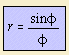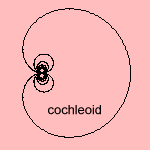# cochleoid

## spiral

last updated: 2004-09-18This cochleoid 1) has been named to the snail 2) by Benthan and Falkenburg (in 1884).
It can be constructed as follows: given a point O and the y-axis. For all circles through O (so tangent to the y-axis), pace a constant distance on the circle. The collection of those points is the cochleoid 3).
Another name for the cochleoid is the

Some relations with other curves are the following:

The first mathematicians to study the curve were J. Peck (1700) and Bernoulli (1726).

notes

1) In Italian: cocleoide.

2) Kochlias (Gr.) = snail.

3) This can be proven as follows: let the circle have a radius t, and center C(t, 0). Now we know that the length of the arc has to be equal to a constant, say a, what is the same as φ t. With sin φ = y / t, this leads to t arcsin(y/t) = a (1).
Now a point of the curve is on the circle, so that (x-t)2 + y2 = t2 and y = 2 r2/2x (2).
Combination of (1) and (2) leads to the desired formula.